# Math operations

Summary of the Math Operations components available in Schematic Editor.

This sub-category provides a wide range of math operation components, such as gain, sum, abs, limit, round, etc. Their descriptions and supported features are given in Table 1.

Table 1. Math operation components in the Signal Processing category of the Schematic Editor core library
Component Description Features Help file
supported input types supported output types vector support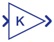Gain

Element-wise gain (y = K.*u) or matrix gain (y = K*u) real, int, uint real, int, uint yes Gain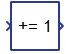Offset

Adds a constant to the input signal of the component. real, int, uint real, int, uint yes Offset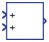Sum*

Add or subtract input signals. real, int, uint real, int, uint yes Sum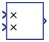Product*

Multiply or divide input signals. real, int, uint real, int, uint yes Product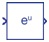Mathematical Function

Mathematical function (exponential, log10, ln, power, sqrt, or reciprocal) real, int, uint real yes Mathematical Function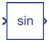Trigonometric Function

Computes a trigonometric function (sin, cos, tan, asin, acos, atan, or atan2) real, int, uint real yes Trigonometric Function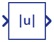Abs

Absolute value of input signal. real, int, uint real, int, uint yes Abs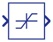Limit

Limit input signal to the upper and lower saturation values. real, int, uint real, int, uint yes Limit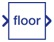Round

Rounds a floating point value to an integer (floor, ceil, round, or fixed) real, int, uint real, int, uint yes Round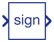Sign

Outputs 1 for positive, -1 for negative, and 0 for 0 input values. real, int, uint real, int, uint yes SignMin Max*

Selects the minimum/maximum value of input signals real, int, uint real, int, uint yes Min Max

*Component supports operation on arbitrary number of input signals.